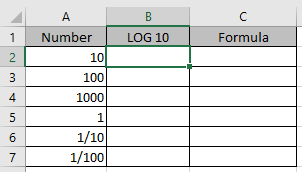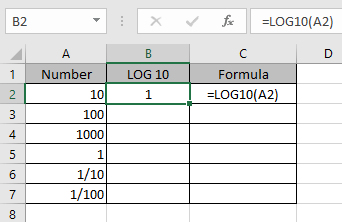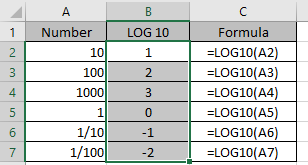# How to use the Excel LOG10 function

In mathematics log 10 is an inverse of an exponential function. The logarithm to base 10 (b = 10) is called the common logarithm.
10x= y
x  = log10(y)

The LOG 10 function returns the log of a value at base 10. For example log(10) at base 10 returns 1.
Syntax:

=LOG10(number)

Note: it works the same as LOG function where base is fixed to 10.

Let’s understand this function using it an example.
Here we have numbers in Column A. we need to find the LOG10 of these numbers.Use the formula

=LOG10(A2)

Here LOG10 returns the Logarithmic value at base 10.Now we will apply the formula to other cells to get the log10 of all numbers.As you can see LOG10 function returns the logarithmic of the numbers at baase 10.

Hope you understood how to use LOG10 function and referring cell in Excel. Explore more articles on Excel mathematical function function here. Please feel free to state your query or feedback for the above article.

Related Article:

LOG function in Excel

Excel LN function

Popular Articles:

How to use the VLOOKUP Function in Excel

How to use the COUNTIF function in Excel 2016

How to Use SUMIF Function in Excel

Terms and Conditions of use

The applications/code on this site are distributed as is and without warranties or liability. In no event shall the owner of the copyrights, or the authors of the applications/code be liable for any loss of profit, any problems or any damage resulting from the use or evaluation of the applications/code.